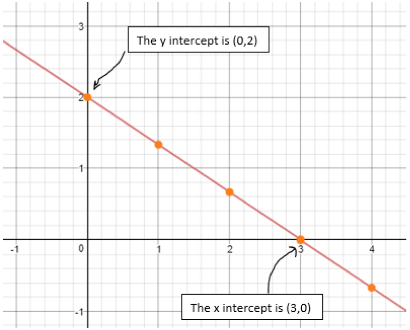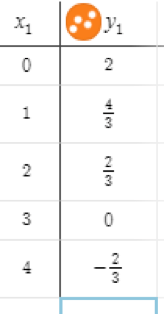# LINR 2 | Lesson 2 | Explore 3

In a graph, the intercepts are the points where a graph crosses the x or y axis.

• For any graph, what do you know about the point where the graph crosses the y-axis?
• For any graph, what do you know about the point where the graph crosses the x-axis?x1 y1 0 2 1 $$\large \frac{4}{3}$$ 2 $$\large \frac{2}{3}$$ 3 0 4 $$-\large \frac{2}{3}$$

Can you identify the x and y intercepts in this table?

The equation of this line in standard form is: $$2x+3y=6$$
How can you use the equation to find the x and y intercepts?
Jenny was mixing various amounts of red and yellow paints to get a variety of shades of orange as shown in the graph. To make the color redder she uses up to 2 cups of red, to make it yellower she uses up to 3 cups of yellow.
What would the x and y intercepts represent in this situation?
What is the slope of this line?
Can you find it from each of the representations?
What is the slope-intercept form of this line? How can you find the x and y-intercepts using this equation?
In another situation, you are given two points on a line: $$(32,\,12)$$ and $$(4,\,34)$$.
What are the intercepts of this line?
A line has x-intercept $$(15,\,0)$$ and slope $$-\Large \frac{3}{5}$$.
Find an equation for this line.